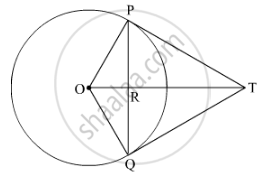Share

# From a point T outside a circle of centre O, tangents TP and TQ are drawn to the circle. Prove that OT is the right bisector of line segment PQ. - CBSE Class 10 - Mathematics

ConceptCircles Examples and Solutions

#### Question

From a point T outside a circle of centre O, tangents TP and TQ are drawn to the circle. Prove that OT is the right bisector of line segment PQ.

#### SolutionTP and TQ are tangents drawn from an external point T to the circle. O is the centre of the circle.

Suppose OT intersect PQ at point R.

In ∆OPT and ∆OQT,

OP = OQ      (Radii of the circle)

TP = TQ       (Lengths of tangents drawn from an external point to a circle are equal.)

OT = OT       (Common sides)

∴ ∆OPT ≅ ∆OQT  (By SSS congruence rule)

So, PTO = QTO    (By CPCT)      .....(1)

Now, in ∆PRT and ∆QRT,

TP = TQ                  (Lengths of tangents drawn from an external point to a circle are equal.)

PTO = QTO       [From (1)]

RT = RT                  (Common sides)

∴ ∆PRT ≅ ∆QRT    (By SAS congruence rule)

So, PR = QR      .....(2)        (By CPCT)

And, PRT = QRT    (By CPCT)

Now,

PRT + QRT = 180°         (Linear pair)

⇒ 2PRT = 180°

PRT = 90°

PRT = QRT = 90°   .....(3)

From (2) and (3), we can conclude that

OT is the right bisector of the line segment PQ.

Is there an error in this question or solution?

#### Video TutorialsVIEW ALL 

Solution From a point T outside a circle of centre O, tangents TP and TQ are drawn to the circle. Prove that OT is the right bisector of line segment PQ. Concept: Circles Examples and Solutions.
S